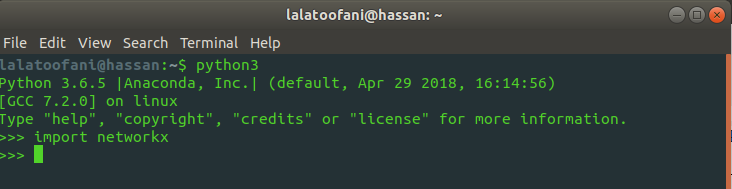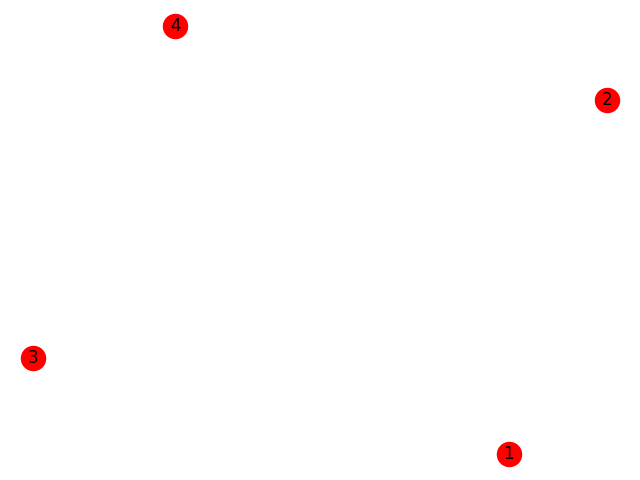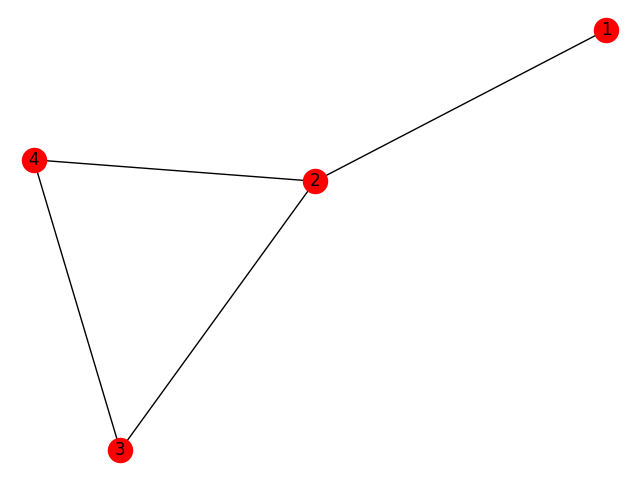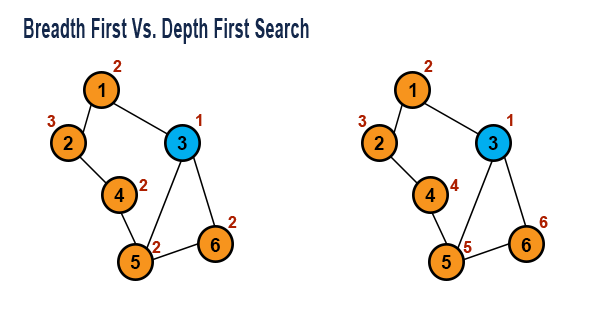## Python Tutorials: How to use Graphic Data Structures in Python

##### Python Tutorials: Learn Python Graphic Data Structures in Detail with Real Life Examples
Written by |19-Oct-2020 | 0 Comments | 416 Views

As we have studied earlier that python doesn’t have many practical modules to run a tree data structure. The binary tree is not that efficient like it has no lookup function. The insert function is also not so proper. The user has to insert manually or by making a list first. However, now what we are going to study is a very vast module. It is very favorable by the developer as it not only solves the problem but also show it visually.

The last data structure that we are going to discuss is graph data structures.

Graph Data Structures:

A graph is a nonlinear data structure consisting of nodes and edges. The nodes are the point that contains the value. Nodes are also known as vertices. The edges connect nodes. Edges are the lines that connect the two nodes, unlike the tree where one node can only connect to two leaf nodes. In a graph, one node can be connected to multiple nodes.

In python, there is a beautiful module to handle this type of data structure. NetworkX is a python module that controls the graph data structure. It is efficient and very well equipped. Not only it solves the problem, but it also visualizes the nodes to make it easy to understand.

So, let’s start by practically doing and solving the problem with networkX.

Installation of NetworkX:

To install, we have to download it by using the pip command, as we always do to download any module in python. However, along with networkX, we also have to download Matplotlib. Matplotlib is a python library to plot data. We will do a detailed analysis of Matplotlib in the future, but here we have to download Matplotlib in python:

 Window User pip install matplotlib pip install networkx   Linux User   sudo pip3 install matplotlib sudo pip3 install networkx

After the easy installation, try to import the networkx in the terminal. If it does not give any particular error, then the networkx is downloaded and ready to use. As given below in figure 1:Figure 1

# Hello to NetworkX:

In this section, we will create an underlying networkX graph and will plot it on a graph. A simple graph will take a few nodes and few edges to connect them with the nodes. Following is the example of basic networkx code:

In the above-given code, we have first import Matplotlib, which is a plotting library in python, and at the second, we import networkx as nx. Nx is used as a short form, so we don’t have to write full name again and again. Then we initialize an empty graph. In that graph, we add four nodes.

In last, we call the draw function of networkx and, in last use, Matplotlib to show the graph. Without Matplotlib, there will be no visualization of the node. The following output is generated as a result of the above-given code:As we can see, there are four nodes in an image. In the networkx draw function, we set with_label attribute equal to true that mark the nodes with a name to make it easy to recognize that which node is which.

Creating Edges:

Now, as we have created nodes. Now we will be able to create the edges. Edges are the connection between nodes. One node may have multiple connections. There is no limitation of nodes in the case of a graph. Following is the code to make the edges in networkX.

We have made an addition to the previous code by making edges in the graph. Following is the output generated by the networkx as a result of the above-given code.From the above output, we can see that four is connected by three, and four is connected by two, making a triangle, and one is connected to two. However, there is a problem. Each connection is an equal length; there is no weight or distance between two nodes. To solve this problem, networkx allows adding weight with the edges.

The above code will set the additional parameters in networkx. It is used to find the shortest path, which we will discuss later in this section.

Add edges and nodes as list:

The above mention code can also be done quickly by giving the list to the nodes and edges. Following is the method to add the nodes and edges using the list data structure in networkx.

 import matplotlib.pyplot as plt import networkx as nx   G = nx.Graph() G.add_nodes_from ([1,2,3,4]) G.add_edges_from ([(1,2), (2,3), (3,4), (4,2)])   nx.draw (G, with_labels=True) plt.show() It will output the same result as we have seen earlier.

Saving the Graph:

We can also save the graph locally on our computer by using the Matplotlib save function. It will save the image in the directory where the program is residing. The following technique is used to save the image:

Different types of graphs:

Graph:

It implements the undirected graph. It ignores multiple edges between two nodes but allows self-looping between two nodes. Following is the method to initialize the graph:

G = nx.Graph()

Di-Graph:

Di-Graph is the graph with the directed edges. It provides the functionality of the directed graph. Following is the method to initialize digraph:

G = nx.DiGraph()

Multigraph:

Multigraph is the graph with undirected edges. It is flexible and allows multiple edges between two nodes. The following method initialized in a multigraph:

G = nx.MultiGraph()

Multidigraph:

Multidigraph is the graph version with directed edges. Following is the method to initialize multi digraph:

G = nx.MultiDiGraph()

Now the question arises how networkX works. What is the functionality behind it? There is no rocket science behind networkx. The building unit of creating a graph in networkx is python dictionaries. The graph in networkx is of dictionaries. The nested dictionaries are created to make the relationship of each dictionary key with others, which collectively form to be a graph data structure.

We can also check how much dictionaries are involved and what is the pattern or schema that is involved in making a graph structure by this simple function as follows:

 import matplotlib.pyplot as plt import networkx as nx   G = nx.Graph() G.add_nodes_from ([1,2,3,4]) G.add_edges_from ([(1,2), (2,3), (3,4), (4,2)])   print (G.adj) Output {1: {2: {}}, 2: {1: {}, 3: {}, 4: {}}, 3: {2: {}, 4: {}}, 4: {3: {}, 2: {}}}

The output is quite complicated, but if we pay a little attention, we can see the pattern that one is associated with only 2 and 2 has a dictionary in which 1,3 and 4 are the keys. It is the basic building unit for creating a graph data structure, which is quite complicated, but networkx made it easy to use.

Breadth-First Search Vs Depth First Search:Breadth-first and depth-first are the two traversing techniques in the graph data structure. In depth-first search, start from the root node and explore more nodes vertically down the hierarchy. In contrast, the breadth-first technique is a graph traversing algorithm that starts from the root node and searches nodes horizontally in a graph. Both techniques are very much used in traversing the graph. Networkx makes it easy to traverse the graph in just a few lines following is the example by code.

 import networkx as nx   nodes = [1,2,3,4] G = nx.Graph() G.add_nodes_from(nodes) G.add_edges_from ([(1,2), (2,3), (3,4), (4,2)])   print (list (nx.dfs_preorder_nodes (G,1, depth_limit=len(nodes)))) Output [1,2,3,4]   Code2 import networkx as nx   nodes = [1,2,3,4] G = nx.Graph() G.add_nodes_from(nodes) G.add_edges_from ([(1,2), (2,3), (3,4), (4,2)])   print (list (nx.dfs_postorder_nodes (G,1, depth_limit=len(nodes)))) Output [4,3,2,1]

The above example is of depth-first search traversal by postorder and pre-order. It is the same post order and pre-order method that we have learned about in tree I data structures.

 import networkx as nx   nodes = [1,2,3,4] G = nx.Graph() G.add_nodes_from(nodes) G.add_edges_from ([(1,2), (2,3), (3,4), (4,2)])   print(list(nx.bfs_predecessors(G,1))) Output [(2, 1), (3, 2), (4, 2)]

Breadth-first is another technique to traverse and find the path of the graph. The output shows the traversal path.

Some Built-in Function in NetworkX:

 import matplotlib.pyplot as plt import networkx as nx   G = nx.Graph() G.add_nodes_from ([1,2,3,4])   nx.draw(G, with_labels=True) plt.show()

 import matplotlib.pyplot as plt import networkx as nx   G = nx.Graph() G.add_nodes_from ([1,2,3,4]) G.add_edges_from ([(1,2), (2,3), (3,4), (4,2)]) nx.draw(G, with_labels=True) plt.show()

3. remove_nodes: remove the nodes and delete the edges of that node too

 import networkx as nx   G = nx.Graph() G.add_nodes_from ([1,2,3,4]) G.add_edges_from ([(1,2), (2,3), (3,4), (4,2)])   G.remove_node (2) print(list(G.nodes)) print(list(G.edges)) Output [1,3,4] [(3,4)]